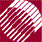ECE532 Biomedical Optics © 1998 Steven L. Jacques, Scott A. Prahl Oregon Graduate Institute

# Time-resolved Monte Carlo

## Launch photons

The first step is to launch a photon. In this example implementation, the photons are launched as an isotropic point source in 3 dimensions bookkeeped using Cartesian coordinates.

• The photon counter is incremented (i_photon)
• The total photon pathlength is initialized to zero (L)
• The time index is zeroed (it)
• The initial photon position is set to the origin (x,y,z)=(0,0,0)
• The initial photon trajectory (ux, uy, uz) is randomized to simulate an isotropic point source. The (ux, uy, uz) values represent the projection of the photon trajectory onto the x, y, and z axes, respectively. The trajectory vector is initially randomized in spherical cooordinates (theta, psi) then converted into the Cartesian coordinate vector (ux, uy, uz).
```/**** LAUNCH
*   Initialize photon position and trajectory.
*   Implements an isotropic point source.
*****/
i_photon += 1;	            /* increment photon count */

photon_status = ALIVE;      /* Launch an ALIVE photon */
L = 0;                      /* set photon's accumulated pathlength to zero */
it = 0;                     /* set pointer to timepoint T */

x = 0;                      /* Set photon position to origin. */
y = 0;
z = 0;

/* Randomly set photon trajectory to yield an isotropic source. */
costheta = 2.0*RandomNum - 1.0;
sintheta = sqrt(1.0 - costheta*costheta);	/* sintheta is always positive */
psi = 2.0*PI*RandomNum;
ux = sintheta*cos(psi);
uy = sintheta*sin(psi);
uz = costheta;
```

NextMonte Carlo